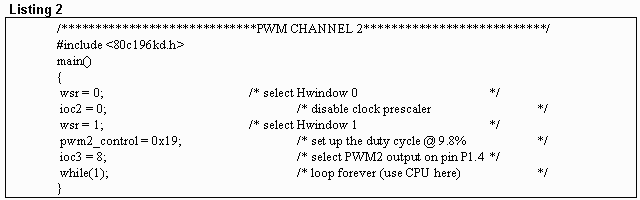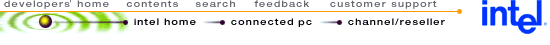# MCS(R) 96 Microcontollers: Back to Basics: Pulse Width Modulator on the 8XC196KC/KD

For additional information about the Pulse Width Modulator, refer to the 8XC196KC/KD User's Manual (order #272238) or ApBUILDER software (order #272216).

The Pulse Width Modulator (PWM) is a very useful peripheral that outputs a variable duty cycle at a fixed frequency. The PWM is very useful in applications like wave generation, motor control and anti-lock braking systems. With the aid of a filter, smooth analog waves can be generated. (See page 10-5 of the 8XC196KC/KD User's Manual). When we filter the output of the PWM, we essentially integrate the area beneath the square wave. This produces an analog output which can simulate a digital- to-analog (D/A) converter.

PWM Functionality
The 8XC196KC/KD is capable of generating up to 3 PWM outputs. The duty cycle can be modified by loading new values into the Special Function Register (SFR) PWMx_CONTROL. The frequency of the PWM can be chosen from 2 frequencies, based on the oscillator frequency. The frequency is selected by loading bit 2 of IOC2 with a 1 or a 0. This peripheral is very handy, because the CPU is not burdened with the task of generating the PWM. This function is solely the task of the PWM modules.

Registers Involved with the PWM
There are only a few SFRs that are necessary to program the Pulse Width Modulator. In this text the key PWM SFRs will be described in detail.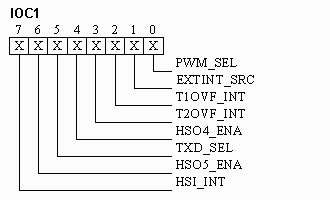In program listings 1 and 2, IOC1 is omitted because we will not be using PWM 0. If we did want to use PWM 0 we would need to set bit 0 to enable the PWM 0 as an output. In this discussion bit 0 is the only one with which we need to concern ourselves.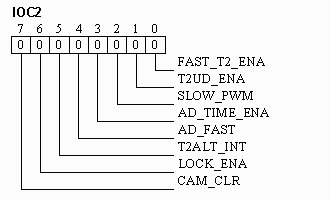In program listings 1 and 2, IOC2 is loaded with 0H. When bit 2 is written as a 1 then the divide-by-2 prescaler is enabled. Essentially this causes the frequency of the PWM output to be halved. For our purposes bit 2 is written as a 0 so we will be using the faster of the two frequencies available. The only bit we need to concern ourselves with is bit 2.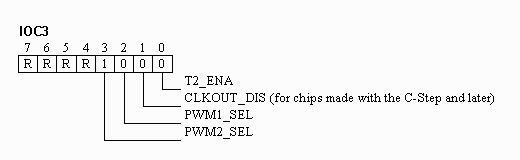The IOC3 SFR is used in program listings 1 and 2 to determine which PWM output we will choose. In order to read or write to this register we need to select Hwindow 1. By placing a 1 in bit 3, PWM2 is selected. PWM2 is selected for a special reason which will be explained later in the text. Bits 4-7 are reserved. All other bits besides bit 3 do not concern us in this discussion.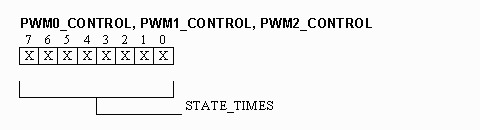The PWMx_CONTROL registers are responsible for modifying the duty cycle. In program listings 1 and 2 PWM2_CONTROL is loaded with 19 hexadecimal or 25 decimal. The maximum value that may be entered into PWMx_CONTROL is FF hexadecimal or 255 decimal. The duty cycle can be calculated by dividing 255 into 25. This yields a duty cycle of 9.8%.

Programming the PWM
Let's take a look at some of the mathematics behind the square wave the PWM is generating. If IOC2 bit 2 is disabled the PWM period = [512/crystal frequency (MHz)]ms. If bit 2 is enabled the PWM period = [1024/crystal frequency (MHz)]ms. Assuming we have a 20MHz crystal and IOC2 bit 2 is disabled the PWM period equals [512/20 MHz]ms or 25.6ms. If IOC2 bit 2 is disabled the high time = [(PWMx_CONTROL * 2) / crystal frequency (MHz)]ms. If bit 2 is enabled the high time = [(PWMx_CONTROL * 4) / crystal frequency (MHz)]ms. Assuming that we have a 20MHz crystal and IOC2 bit 2 is disabled the high time equals [(25 * 2) / 20 MHz] or 2.5ms. We can also calculate the low time by subtracting the high time from the period. This equals [25.6ms - 2.5ms] or 23.1ms. (See page 10-2 and 10-3 of the 8XC196KC/KD User's Manual for additional information on wave characteristics.) If still more rigorous PWM specifications are required, see the Programming the HSO FaxBack in this series.

Now lets take a look at some key points to programming the PWM. In the previous text it was noted that PWM2_CONTROL was chosen for a reason. NOTE: Depending on which include file you have the PWM2_CONTROL register is not correctly initialized. Check the file 80C196KD.INC to see that PWM2_CONTROL equals 17H. Some include files have PWM2_CONTROL set to 16H, which is incorrect.